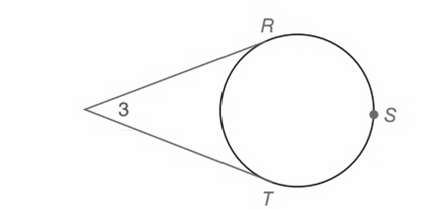Chapter 6.CT, Problem 7CT### Elementary Geometry for College St...

6th Edition
Daniel C. Alexander + 1 other
ISBN: 9781285195698

#### Solutions

Chapter
Section### Elementary Geometry for College St...

6th Edition
Daniel C. Alexander + 1 other
ISBN: 9781285195698
Textbook Problem
1 views

# Given the tangents with m ∠ 3 = 46 ° , find:a) m R S T ⌢                                   _ b) m R T ⌢                                   _To determine

(a)

To find:

mRST.

Explanation

Theorem used:

The measure of an angle formed when two secants intersect at a point outside the circle is one-half the difference of the measure of the two intercepts arcs.

Given:

m3=46°.

Calculation:

Consider the figure,

From the theorem we know that,

m3=mRSTmRT2(1).

Let us consider, mRST=x°.

And mRT=360°x°

Substitute for mRST and mRT in (1) we have,

To determine

(b)

To find:

mRT.

### Still sussing out bartleby?

Check out a sample textbook solution.

See a sample solution

#### The Solution to Your Study Problems

Bartleby provides explanations to thousands of textbook problems written by our experts, many with advanced degrees!

Get Started

#### Find the limit or show that it does not exist. limxx+3x24x1

Single Variable Calculus: Early Transcendentals, Volume I

#### In Exercises 23-36, find the domain of the function. 25. f(x)=3x+1x2

Applied Calculus for the Managerial, Life, and Social Sciences: A Brief Approach

#### For y = x sin x, y = _____. a) x cos x b) x cos x + 1 c) cos x d) x cos x + sin x

Study Guide for Stewart's Single Variable Calculus: Early Transcendentals, 8th